### SOLUTIONS TO DIFFERENTIATION OF INVERSE TRIGONOMETRIC FUNCTIONS

SOLUTION 1 : Differentiate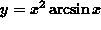. Apply the product rule. Then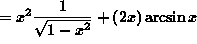(Factor an x from each term.).

SOLUTION 2 : Differentiate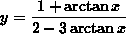. Apply the quotient rule. Then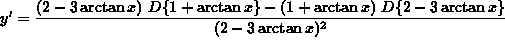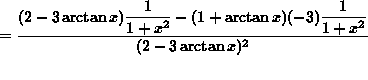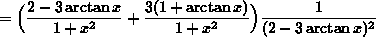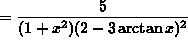.

SOLUTION 3 : Differentiate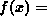arcarc. Apply the product rule. Thenarcarc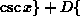arcarcarc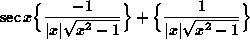arc= ( arc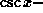arc.

SOLUTION 4 : Letarc. Solve f'(x) = 0 for x . Begin by differentiating f . Then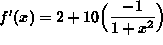(Get a common denominator and subtract fractions.)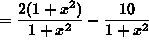.

(It is a fact that if, then A = 0 .) Thus,

2(x - 2)(x+2) = 0 .

(It is a fact that if AB = 0 , then A = 0 or B=0 .) It follows that

x-2 = 0 or x+2 = 0 ,

that is, the only solutions to f'(x) = 0 are

x = 2 or x = -2 .

SOLUTION 5 : Let. Show that f'(x) = 0 . Conclude that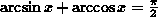. Begin by differentiating f . Then.

If f'(x) = 0 for all admissable values of x , then f must be a constant function, i.e.,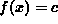for all admissable values of x ,

i.e.,for all admissable values of x .

In particular, if x = 0 , then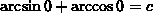i.e.,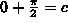.

Thus,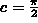andfor all admissable values of x .

SOLUTION 6 : Evaluate. It may not be obvious, but this problem can be viewed as a derivative problem. Recall that(Since h approaches 0 from either side of 0, h can be either a positve or a negative number. In addition,is equivalent to. This explains the following equivalent variations in the limit definition of the derivative.).

If, then, and letting, it follows that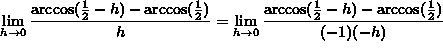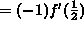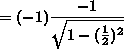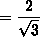.

The following problems require use of the chain rule.

SOLUTION 7 : Differentiate. Use the product rule first. Then(Apply the chain rule in the first summand.)(Factor out. Then get a common denominator and add.)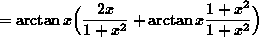.

SOLUTION 8 : Differentiate. Apply the chain rule twice. Then(Recall that.)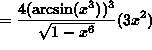.

SOLUTION 9 : Differentiate. Apply the chain rule twice. Then(Recall that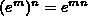.)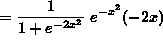.

SOLUTION 10 : Determine the equation of the line tangent to the graph ofat x = e . If x = e , then, so that the line passes through the point. The slope of the tangent line follows from the derivative (Apply the chain rule.)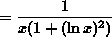.

The slope of the line tangent to the graph at x = e is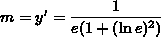.

Thus, an equation of the tangent line is.